In :
%pylab inline
rc('text', usetex=True)
rc('axes', labelsize=15, titlesize=15)

Populating the interactive namespace from numpy and matplotlib

In :
import seaborn as sns
sns.set_style("white")
np.random.seed(10)


# ABC PMC on a 2D gaussian example¶

In this example we're looking at a dataset that has been drawn from a 2D gaussian distribution. We're going to assume that we don't have a proper likelihood but that we know the covariance matrix $\Sigma$ of the distribution. Using the ABC PMC algorithm we will approximate the posterior of the distribtion of the mean values.

First we generate a new dataset by drawing random variables from a mulitvariate gaussian around mean=[1.1, 1.5]. This is going to be our observed data set

In :
samples_size = 1000
sigma = np.eye(2) * 0.25
means = [1.1, 1.5]

data = np.random.multivariate_normal(means, sigma, samples_size)
matshow(sigma)
title("covariance matrix sigma")
colorbar()

Out:
<matplotlib.colorbar.Colorbar instance at 0x10c542950>
/Users/jakeret/Library/Python/2.7/lib/python/site-packages/matplotlib/collections.py:590: FutureWarning: elementwise comparison failed; returning scalar instead, but in the future will perform elementwise comparison
if self._edgecolors == str('face'):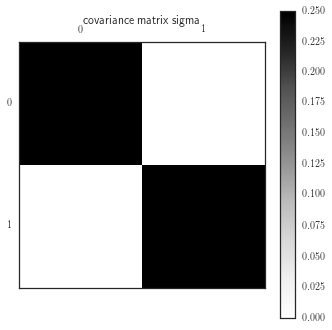Then we need to define our model/simulation. In this case this is simple: we draw again random variables from a multivariate gaussian distribution using the given mean and the sigma from above

In :
def create_new_sample(theta):
return np.random.multivariate_normal(theta, sigma, samples_size)


Next, we need to define a distance measure. We will use the sum of the absolute differences of the means of the simulated and the observed data

In :
def dist_measure(x, y):
return np.sum(np.abs(np.mean(x, axis=0) - np.mean(y, axis=0)))


### Verification¶

To verify if everything works and to see the effect of the random samples in the simulation we compute the distance for 1000 simulations at the true mean values

In :
distances = [dist_measure(data, create_new_sample(means)) for _ in range(1000)]

In :
sns.distplot(distances, axlabel="distances", )
title("Variablility of distance from simulations")

Out:
<matplotlib.text.Text at 0x10c7317d0>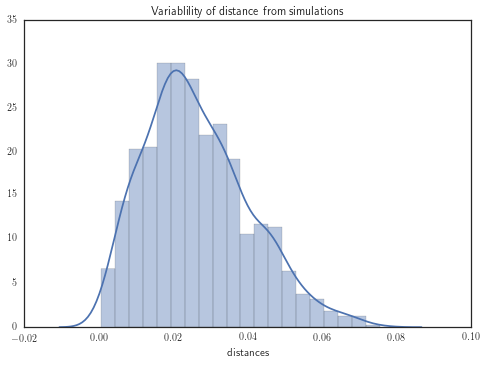### Setup¶

Now we're going to set up the ABC PMC sampling

In :
import abcpmc


As a prior we're going to use a gaussian prior using our best guess about the distribution of the means.

In :
prior = abcpmc.GaussianPrior(mu=[1.0, 1.0], sigma=np.eye(2) * 0.5)


As threshold $\epsilon$ we're going to use the $\alpha^{th}$ percentile of the sorted distances of the particles of the current iteration. The simplest way to do this is to define a constant $\epsilon$ and iteratively adapt the theshold. As starting value we're going to define a sufficiently high value so that the acceptance ratio is reasonable and we will sample for T iterations

In :
alpha = 75
T = 20
eps_start = 1.0
eps = abcpmc.ConstEps(T, eps_start)


Finally, we create an instance of your sampler. We want to use 5000 particles and the functions we defined above. Additionally we're going to make use of the built-in parallelization and use 7 cores for the sampling

In :
sampler = abcpmc.Sampler(N=5000, Y=data, postfn=create_new_sample, dist=dist_measure, threads=7)


Optionally, we can customize the proposal creation. Here we're going to use a "Optimal Local Covariance Matrix"-kernel (OLCM) as proposed by (Fillipi et al. 2012). This has shown to yield a high acceptance ratio togheter with a faster decrease of the thresold.

In :
sampler.particle_proposal_cls = abcpmc.OLCMParticleProposal


### Sampling¶

Now we're ready to sample. All we need to do is to iterate over the yielded values of your sampler instance. The sample function returns a namedtuple per iteration that contains all the information that we're interestend in

In :
def launch():
eps = abcpmc.ConstEps(T, eps_start)

pools = []
for pool in sampler.sample(prior, eps):
print("T: {0}, eps: {1:>.4f}, ratio: {2:>.4f}".format(pool.t, eps(pool.eps), pool.ratio))

for i, (mean, std) in enumerate(zip(*abcpmc.weighted_avg_and_std(pool.thetas, pool.ws, axis=0))):
print(u"    theta[{0}]: {1:>.4f} \u00B1 {2:>.4f}".format(i, mean,std))

eps.eps = np.percentile(pool.dists, alpha) # reduce eps value
pools.append(pool)
sampler.close()
return pools

In :
import time
t0 = time.time()
pools = launch()
print "took", (time.time() - t0)

T: 0, eps: 1.0000, ratio: 0.3907
theta: 1.0648 ± 0.3761
theta: 1.3485 ± 0.3746
T: 1, eps: 0.8351, ratio: 0.5106
theta: 1.0694 ± 0.2965
theta: 1.3742 ± 0.2963
T: 2, eps: 0.6666, ratio: 0.5363
theta: 1.0802 ± 0.2421
theta: 1.4044 ± 0.2410
T: 3, eps: 0.5282, ratio: 0.5230
theta: 1.0808 ± 0.1926
theta: 1.4349 ± 0.1924
T: 4, eps: 0.4158, ratio: 0.5268
theta: 1.0863 ± 0.1570
theta: 1.4595 ± 0.1524
T: 5, eps: 0.3299, ratio: 0.5270
theta: 1.0855 ± 0.1257
theta: 1.4696 ± 0.1248
T: 6, eps: 0.2624, ratio: 0.4958
theta: 1.0926 ± 0.0993
theta: 1.4822 ± 0.0999
T: 7, eps: 0.2085, ratio: 0.5031
theta: 1.0904 ± 0.0780
theta: 1.4843 ± 0.0805
T: 8, eps: 0.1663, ratio: 0.5006
theta: 1.0906 ± 0.0633
theta: 1.4885 ± 0.0652
T: 9, eps: 0.1322, ratio: 0.4851
theta: 1.0905 ± 0.0520
theta: 1.4903 ± 0.0516
T: 10, eps: 0.1062, ratio: 0.4743
theta: 1.0919 ± 0.0430
theta: 1.4917 ± 0.0417
T: 11, eps: 0.0846, ratio: 0.4652
theta: 1.0915 ± 0.0346
theta: 1.4931 ± 0.0347
T: 12, eps: 0.0683, ratio: 0.4381
theta: 1.0920 ± 0.0286
theta: 1.4931 ± 0.0290
T: 13, eps: 0.0563, ratio: 0.4155
theta: 1.0923 ± 0.0250
theta: 1.4943 ± 0.0254
T: 14, eps: 0.0463, ratio: 0.3605
theta: 1.0920 ± 0.0224
theta: 1.4944 ± 0.0219
T: 15, eps: 0.0386, ratio: 0.3187
theta: 1.0924 ± 0.0200
theta: 1.4945 ± 0.0193
T: 16, eps: 0.0319, ratio: 0.2739
theta: 1.0919 ± 0.0181
theta: 1.4948 ± 0.0179
T: 17, eps: 0.0271, ratio: 0.2259
theta: 1.0917 ± 0.0174
theta: 1.4946 ± 0.0166
T: 18, eps: 0.0231, ratio: 0.1805
theta: 1.0917 ± 0.0164
theta: 1.4948 ± 0.0159
T: 19, eps: 0.0197, ratio: 0.1425
theta: 1.0922 ± 0.0157
theta: 1.4949 ± 0.0158
took 149.579810143


### Postprocessing¶

How did the sampled values evolve over the iterations? As the threshold is decreasing we expect the errors to shrink while the means converge to the true means.

In :
for i in range(len(means)):
moments = np.array([abcpmc.weighted_avg_and_std(pool.thetas[:,i], pool.ws, axis=0) for pool in pools])
errorbar(range(T), moments[:, 0], moments[:, 1])
hlines(means, 0, T, linestyle="dotted", linewidth=0.7)
_ = xlim([-.5, T])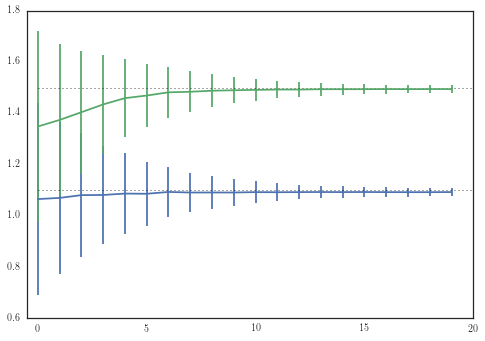How does the distribution of the distances look like after we have approximated the posterior? If we're close to the true posterior we expect to have a high bin count around the values we've found in the earlier distribution plot

In :
distances = np.array([pool.dists for pool in pools]).flatten()
sns.distplot(distances, axlabel="distance")

Out:
<matplotlib.axes._subplots.AxesSubplot at 0x10c5682d0>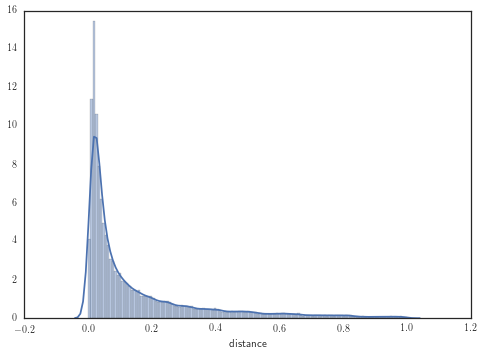How did our $\epsilon$ values behave over the iterations? Using the $\alpha^{th}$ percentile causes the threshold to decrease relatively fast in the beginning and to plateau later on

In :
eps_values = np.array([pool.eps for pool in pools])
plot(eps_values, label=r"$\epsilon$ values")
xlabel("Iteration")
ylabel(r"$\epsilon$")
legend(loc="best")

Out:
<matplotlib.legend.Legend at 0x10d769110>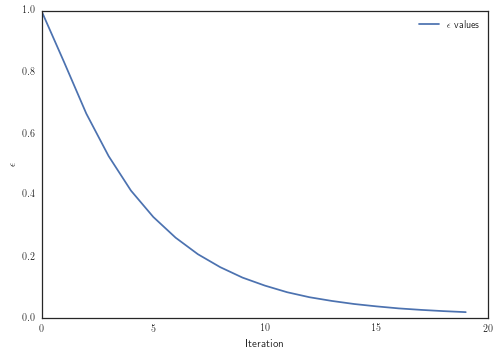What about the acceptance ratio? ABC PMC with the OLCM kernel gives us a relatively high acceptance ratio.

In :
acc_ratios = np.array([pool.ratio for pool in pools])
plot(acc_ratios, label="Acceptance ratio")
ylim([0, 1])
xlabel("Iteration")
ylabel("Acceptance ratio")
legend(loc="best")

Out:
<matplotlib.legend.Legend at 0x10cc2e210>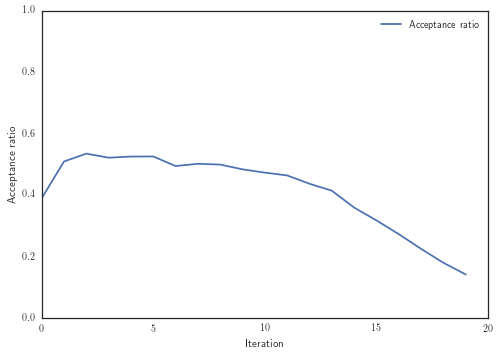In :
%pylab inline
rc('text', usetex=True)
rc('axes', labelsize=15, titlesize=15)

Populating the interactive namespace from numpy and matplotlib


Finally what does our posterior look like? For the visualization we're using triangle.py (https://github.com/dfm/triangle.py)

In :
import triangle
samples = np.vstack([pool.thetas for pool in pools])
fig = triangle.corner(samples, truths= means)

/Users/jakeret/Library/Python/2.7/lib/python/site-packages/matplotlib/collections.py:650: FutureWarning: elementwise comparison failed; returning scalar instead, but in the future will perform elementwise comparison
if self._edgecolors_original != str('face'):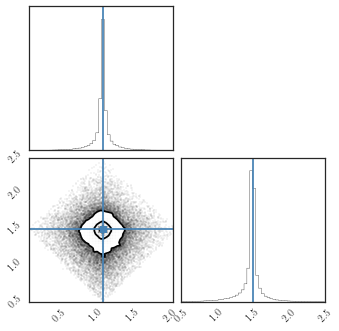Omitting the first couple of iterations..

In :
idx = -1
samples = pools[idx].thetas
fig = triangle.corner(samples, weights=pools[idx].ws, truths= means)

for mean, std in zip(*abcpmc.weighted_avg_and_std(samples, pools[idx].ws, axis=0)):
print(u"mean: {0:>.4f} \u00B1 {1:>.4f}".format(mean,std))

mean: 1.0922 ± 0.0157
mean: 1.4949 ± 0.0158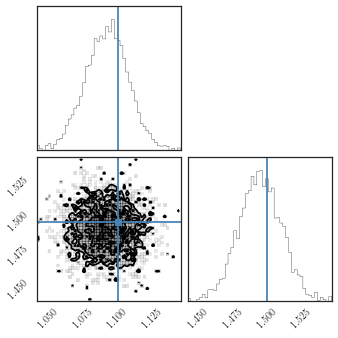In [ ]: